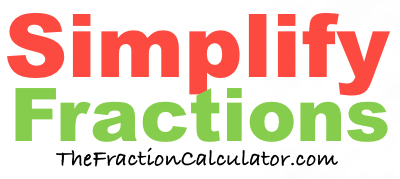What is 48/52 simplified?Here we will simplify 48/52 to its simplest form and convert it to a mixed number if necessary.

In the fraction 48/52, 48 is the numerator and 52 is the denominator.

When you ask "What is 48/52 simplified?", we assume you want to know how to simplify the numerator and denominator to their smallest values, while still keeping the same value of the fraction.

We do this by first finding the greatest common factor of 48 and 52, which is 4.

Then, we divide both 48 and 52 by the greatest common factor to get the following simplified fraction:

12/13

Therefore, this equation is true:

48/52 = 12/13

If the numerator is greater than or equal to the denominator of a fraction, then it is called an improper fraction. In that case, you could convert it into a whole number or mixed number fraction.

12/13 = Proper Fraction

Simplify Fractions
Here you can submit another fraction for us to simplify:

/

What is 48/53 simplified?
Here is the next fraction on our list that we simplified.